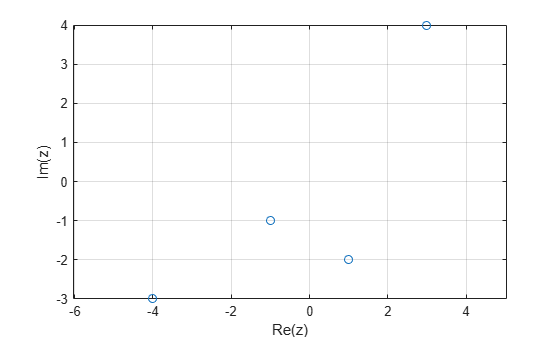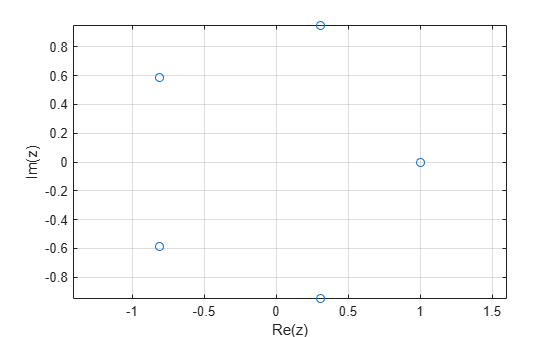# Plot Imaginary and Complex Data

### Plot One Complex Input

This example shows how to plot the imaginary part versus the real part of a complex vector, `z`. With complex inputs, `plot(z)` is equivalent to `plot(real(z),imag(z))`, where `real(z)` is the real part of `z` and `imag(z)` is the imaginary part of `z`.

Define `z` as a vector of eigenvalues of a random matrix.

`z = eig(randn(20));`

Plot the imaginary part of `z` versus the real part of `z`. Display a circle at each data point.

```figure plot(z,'o')```### Plot Multiple Complex Inputs

This example shows how to plot the imaginary part versus the real part of two complex vectors, `z1` and `z2`. If you pass multiple complex arguments to `plot`, such as `plot(z1,z2)`, then MATLAB® ignores the imaginary parts of the inputs and plots the real parts. To plot the real part versus the imaginary part for multiple complex inputs, you must explicitly pass the real parts and the imaginary parts to `plot`.

Define the complex data.

```x = -2:0.25:2; z1 = x.^exp(-x.^2); z2 = 2*x.^exp(-x.^2);```

Find the real part and imaginary part of each vector using the `real` and `imag` functions. Then, plot the data.

```real_z1 = real(z1); imag_z1 = imag(z1); real_z2 = real(z2); imag_z2 = imag(z2); plot(real_z1,imag_z1,'g*',real_z2,imag_z2,'bo')```